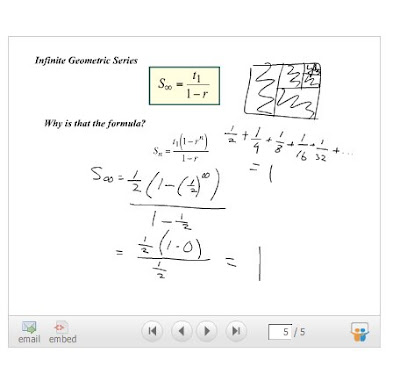Wednesday, June 4, 2008

Infinite Geometric Series

OVERVIEW:

• Finding the Sum of Numbers in a Sequence
• Sigma Notation
• Infinite Geometric Series Formula

Finding the Sum of Numbers in a Sequence(a) What is the sum of the integers from 1 to 5000?

Credit to Paul for being our Gauss today.

We imagine the sequence of all the numbers 1 to 5000 in the top row while the bottom row has all the numbers from 5000 to 1.

Example:
1, 2, 3, ... 5000
5000, 4999, 4998, ... 1

We find the sum of each column in our table and see that they're all 5001. Refer to slide 4 of June 3's slide for a better understanding of what I'm talking about.

We know that there are 5000 of these terms. We also know that if we want to find the sum of the integers between 1 to 5000, then we would have to multiply the number of terms by the total we have then divide by 2. We divided by 2 because there were two sequences we added.

Example: 5000 * 5001 / 2 = 12 502 500

Another way of solving this problem is to pull out the equation (found on June 3's slide #7) and plugging in the appropriate numbers, which Mr. K has a distaste for using this method because we're not really understanding the concept but just plugging numbers into an equation.

(b) What is the sum of all multiples of 7 between 1 & 5000?

The lowest multiple of 7 between 1 and 5000 is 7.
The highest multiple of 7 between 1 and 5000 is 4998.
There are 714 terms that are multiples of 7 between 1 and 5000.

We then imagine, again, that table, listing all the numbers in the sequence from 1 to 5000 (that are multiples of 7) in the top row and all the numbers in the sequence from 5000 to 1 (that are multiples of 7) in the bottom row, and find the sum of each column.

Example:
7, 14, 21, ..., 4998
4998, 4991, 4984, ..., 7

Add the top row and the bottom row, and you find that under each column in our imaginary table is 5005.

We multiplied the number of multiples of 7 between 1 and 5000 by the sum of the first and last terms of the sequence and divided that by 2 because we've counted the sequence twice and we get 1 786 785.

(c) What is the sum of all integers from 1 to 5000 inclusive that are not multiples of 7?

Well, we have the sum of all the integers from 1 to 5000. We have the sum of all the multiples of 7 between 1 and 5000. So if we find the difference of those two numbers, we find the sum of all the integers from 1 to 5000 inclusive that are not multiples of 7.

12 502 500 - 1 786 785 = 10 715 715

Sigma NotationLeft Question:
• The k = 1 means that you start with 1 and evaluate 3k.
• The number above the sigma symbol, 4, means to keep evaluating 3k until k = 4, then add all the terms.

Right Question:
• The k = 0 means that you start with 0 and evaluate 2^k.
• The number above the sigma symbol, 3, means to keep evaluating 2^k until k = 3, then add all the terms.

Then Mr. K says to find another sigma notation that is equivalent to the two questions on the slide. Of course, "mathematics is the science of patterns" is the common phrase that Mr. K always says, and yes, there're patterns here.

Also, a quick way of figuring out the answer to the sigma notation in question is to add the first and last term, then multiply by 2. Example: In the left question, [3(1) + 3(4)] * 2 = 30

Infinite Geometric Series FormulaThe formula given in the above slide is something that we already derived from the previous class.

1/2 + 1/4 + 1/8 + 1/16 + 1/32 ... = 1

The formula that we derived from the previous class was

Sn = t1*(1-r^infinity) / (1-r)

If we take r and raise it to the power of infinity, that would equal zero.
Example: r = 1/2

1/2 * 1/2 * 1/2 * 1/2 ... = 0

So, in the part of the formula where it says (1-r^infinity), that part of the formula is going to equal (1-0) = 1 anyways, and multiplying a number by 1 won't really have a visible effect. It's more efficient to just not include the (1-r^infinity) into the formula.

Plug in the numbers into the equation, BING! BANG! BOOM! you have the result. Have a nice day.

END NOTES
• Start on Exercise 47: Infinite Geometric Series. You should have finished all the questions in the exercise book up to 47.
• Tomorrow, we will finish off the lesson the infinite geometric series.
• Hopefully, we will be on task and finish the course tomorrow, and do a review on Friday.
• Next scribe is Francis.